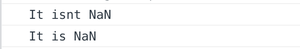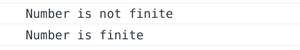# How to check whether a number is NaN or finite in JavaScript ?

• Last Updated : 05 Nov, 2021

In this article, we will see how to check whether number is NaN or finite. To check whether the given number is NaN or finite, we can use JavaScript methods.

1. isNaN() Method: To determine whether a number is NaN, we can use the isNaN() function. It is a boolean function that returns true if a number is NaN otherwise returns false.

Syntax:

`isNan(parameter)`

Example 1:

## Javascript

 ``

Output:Note: The isNaN() function can alternatively be used as Number.isNaN(). It is considered to be more robust version of the original.

Example 2:

## Javascript

 ``

Output:Note: isNaN() (or Number.isNaN()) can be used to deduce if a number is NaN or not but it doesn’t says anything about the number being finite.

2. isFinite() Method: To determine whether a number is finite we can use the isFinite() function. It is a boolean function that returns true if a number is Finite otherwise false.

Syntax:

`isFinite(parameter)`

Example 1:

## Javascript

 ``

output:Example 2:

## Javascript

 ``

Output:Note: If needed, the isFinite() function can parse the parameter into number

Example 3:

## Javascript

 ``

Output:My Personal Notes arrow_drop_up